# Panoptic Segmentation — The Panoptic Quality Metric.

In the previous article, I gave an explanation about various computer vision tasks, ending with Panoptic Segmentation.

If you missed the previous article, you can read it here.

While that article did describe the nature of various computer vision tasks and their value, it missed out a really important element:

How do we measure how well we are performing the task?

So today I would like to take you through a guide of the various performance metrics that are used in computer vision today. Leading you to the Panoptic Quality metric, which is used to assess the Panoptic Segmentation task.

# How do we tell a good prediction from a bad one?

In object detection, we want our algorithm to not only predict what class our object belongs to, but we also want it to identify where in the picture it’s located.

Taking an example of a single cat image, let’s suppose we want our algorithm to identify and locate the cat like so: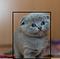Cat with ground truth

The black bounding box is the ‘answer’ that we want our algorithm to predict, which is commonly called the ‘ground truth’. So imagine we let our algorithm make a prediction and it goes ahead and produces the following bounding box: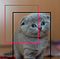Ground truth plus prediction on our cat image.

Is that a good prediction or a bad prediction? We may have subjective opinions on it (I mean, it sort of got half the cat), but we need to have a concrete way to measure it.

# Intersection over Union

A very common way to describe the quality of our prediction is to use the intersection over union equation: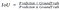Intersection Over Union Equation

If we momentarily remove our cat from the ground truth and prediction, we can visually see what this looks like:

By diving the area the prediction and ground truth intersect by the area they both consume, we get a ratio that is inclusively between 0 and 1, with 0 meaning there is no intersection and 1 being a perfect fit.

We can use the value of this ratio to identify whether or not our algorithm made an accurate prediction or failed to identify the target object. For example, we could say:

A value greater than or equal to 0.5 is a successful prediction and below is unsuccessful.

While a `threshold ≥ 0.5` is a common value, in real world systems this number will be dependent on the task at hand and what quality of bounding box prediction you need. You need to pick the right value for the job you’re doing.

# True Positive, False Negative and False Positive

So what if our prediction has a threshold value above 0.5? Well, we call that a true positive, or TP.

If it’s below? Well then we have two negative events that occur: A false negative and false positive: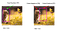TP, FN and FP Example

When the bounding box IoU is below our threshold, as shown in the image above (right side), we conclude that the prediction was not correct, resulting in a situation where:

• We made a prediction that did not contain an object, a false positive.
• We failed to predict the presence of the object, a false negative.

Now that we have a way of determining good and bad predictions, we can combine this knowledge to form some metrics!

# Precision and Recall

Precision could be described with the following:

Out of all the shots I’ve taken, how many have been bulls-eyes?

Or more relevantly to us:

Of all the predictions, how many have been successful?

Expanding on our cat classification task, we can produce a small cat data-set and calculate our precision: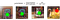Precision calculation for our data-set.

In this example, our precision will be `0.75`, as we got 3 / 4 predictions correct.

Recall on the other-hand, works slightly differently:

Out of all the objects, how many did we detect?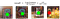Recall calculation for our data-set

In this example, we can see our recall being `0.75` as we were able to recall 3/4 cats.

# Average Precision (AP)

Let’s stick to our 4 kitty data set above.

In order to calculate AP, we must rank our cat predictions based on our algorithms class probability (confidence) scores.

Normally these confidence scores will get produced when your model makes a prediction, but since we don’t have any actual predictions in our dummy data-set, let’s add some fake ones for illustration:Adding Confidence Scores

So to calculate AP, what we do is rank our predictions and then produce an accumulating precision-recall graph.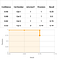Precision-Recall Graph

We calculate precision and recall by looping through our predictions from highest confidence to lowest, assessing the precision and recall for our current moment in time:

1. At the highest ranked prediction (cat 1) we predicted correctly `precision = 1/1 & recall = 1/1`.

2. At the 2nd ranked prediction (cat 3) we predicted correctly `precision = 2/2 & recall = 2/2`.

3. At the 3rd ranked prediction (cat 2) we predicted correctly `precision = 3/3 & recall = 3/3`.

4. At the 4th ranked prediction (cat 4) we predicted incorrectly `precision = 3/4 & recall = 3/4`.

Now that we have our accumulative precision and recall, we can produce the graph shown above where our x axis is recall and the y axis is our precision.

Average precision is then calculated by finding out the area under the graph. One important caveat to note is that this area is measured from a recall position of 0.

In our case, there is no data starting at recall = 0, but we can produce this data by taking the proceeding maximum value of precision.

So what is our proceeding maximum value of precision? Moving from `recall = 0` to `recall = 0.25` our precision value becomes 1. So we can extrapolate that maximum precision value to our `recall = 0` position: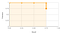Extrapolated for Recall = 0

Now the area under the graph can be calculated as `0.75 * 1 = 0.75`, which is our AP score.

# Mean Average Precision (mAP)

This metric is most commonly used in object detection and instance segmentation. Often it is simply shortened to AP.

The AP calculation that we did above applies to one class, which in our case was a cat data set, but what if we have multiple classes?

Well that’s where mean average precision comes in.

Suppose we have a data set of cats, dogs, cars, bicycles and balloons. What we could do is calculate the AP for all those classes separately and then calculate the mean of our AP scores: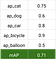mAP, the average of all AP scores.

# Segmentation IoU

So remember when we calculated the bounding box IoU? We can perform the same calculation for segments. This is a commonly used metric for semantic segmentation

In semantic segmentation our image will contain a whole bunch of different classes that we need to predict.

We can isolate our predicted segments and then pair them with their corresponding binary mask ground truths and then perform IoU. Here’s an example for the cat classbelow:

# The Multiple Purposes of IoU

Before carrying on to the Panoptic Quality it’s important to clarify the two ways that IoU is used:

1. Intersection Over Union is used as a way to identify true positives, false positives and false negatives by selecting a threshold value to distinguish them. For the purpose of object detection and instance segmentation, this enables us to then calculate our precision, recall, AP and mAP to assess our model.
2. Intersection Over Union is used as a way to assess the segmentation or bounding box quality. Meaning for each true positive, how good was the segmentation? This is most often used in Semantic Segmentation, where AP cannot be used, due to the lack of clearly defined confidence scores in semantic segmentation predictions.

# Panoptic Quality.

The PQ metric is used to evaluate the performance of our model in Panoptic Segmentation tasks. It looks like this: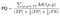The Panoptic Quality

A lot of the symbols should look pretty familiar. The top of the equation is summing up all the Intersection Over Union ratios for all the true positives (TP) values we have. The bottom is some kind of happy blend of precision and recall, where we are dividing all the True Positives and half the False Positives and False Negatives.

An even better way to digest what this metric does is to look at it broken into two sections:

Here we have two sections, segmentation quality and recognition quality.

Segmentation quality ( SQ ) is evaluating how closely matched our segments are with their ground truths. When this value comes closer to 1, it means that our ( TP ) predicted segments are more closely matched with their ground truths. However, it doesn’t take into account any of our bad predictions.

That’s where the recognition quality ( RQ ) comes in. This metric is a combination of precision and recall, attempting to identify how effective our model is at getting a prediction right.

# Why do we need PQ? Why not just use AP or IoU?

In order to calculate AP we require confidence scores so that we can rank our predictions from highest to lowest and then produce our precision / recall graph. Unfortunately for us, as panoptic segmentation combines instance and semantic segmentation together, we have no clear cut confidence scores to speak of for semantic predictions.

Using a basic IoU metric also causes some issues. In semantic segmentation, we only have 1 segment to compare with 1 ground truth per class. However in panoptic segmentation we can have multiple instances of the same class and multiple ground truths:

# Segment Matching

An important part of the PQ metric is that it performs a process called segment matching.

Segment matching solves the issue of matching the correct predicted segment to their corresponding ground truth. It follows two basic principles:

1. No single pixel can belong to two predicted segments at the same time, i.e. no overlapping predictions.
2. A predicted segment can only be ‘matched’ with a ground truth if its IoU with that ground truth is > 0.5.

So lets take an example: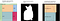Matching a segment with a ground truth label

In our example above, we can see that there are two predictions that are overlaying this one particular ground truth. Because of our two golden rules above, we know that at no point in time can two predicted segments have an IoU with a specific ground truth that is > 0.5. Only one can.

So in our example above we can see that the bottom (blue) cat prediction would be the “matched prediction”, which would leave the top one as a false positive ( FP ).

Once we have our matching segments, we can sum up our TP, FP and FN values, perform our IoU calculations and get our PQ metric!

So that’s it for this article. At this stage you should have a good understanding of the Panoptic Segmentation task and the PQ metric used to assess your model’s results.

If you want to learn more about panoptic segmentation, I would highly recommend you try the Panoptic Segmentation Challenge for yourself. If you need a reference then feel free to check out my submission on github here.

Thanks for reading!

Written by

## Daniel Mechea

#### With a love for engineering, Daniel has developed API’s, ICO’s, websites, machine-learning systems, simulations, robotic systems and electric race-cars.

Welcome to a place where words matter. On Medium, smart voices and original ideas take center stage - with no ads in sight. Watch
Follow all the topics you care about, and we’ll deliver the best stories for you to your homepage and inbox. Explore
Get unlimited access to the best stories on Medium — and support writers while you’re at it. Just \$5/month. Upgrade